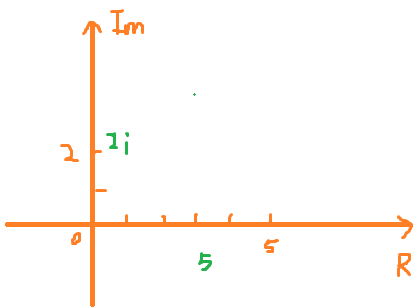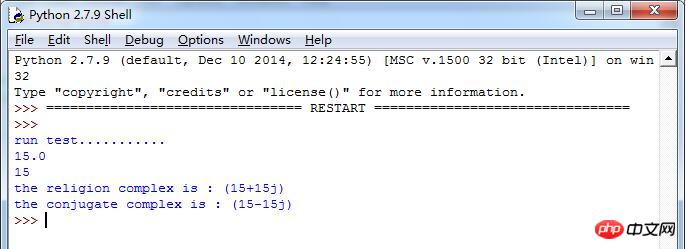• 复数的引入 追根求源，最初是为了求解没有实数根的二次方程。例如求解 x2+1=0x2+1=0x^2+1=0 这个由实数组成的方程，显然没有实数根。 所以复数集可以看成实数集合的一个自然扩充。 首先引入一个“新数”iii。使...
复数的引入

追根求源，最初是为了求解没有实数根的二次方程。例如求解
x2+1=0${x}^{2}+1=0$x^2+1=0
这个由实数组成的方程，显然没有实数根。
所以复数集可以看成实数集合的一个自然扩充。
首先引入一个“新数”i$i$$i$。使它满足
i2=−1${i}^{2}=-1$i^2=-1
也就是说i$i$$i$是x2+1=0${x}^{2}+1=0$x^2+1=0
的解。
我们再给复数定义：
形如z=a+bi$z=a+bi$$z=a+bi$的数就是复数。
其中a$a$$a$和b$b$$b$分别叫做复数z$z$$z$的实部和虚部。
注意，b$b$$b$才是虚部，bi$bi$$bi$不是虚部。
记作：a=Re(z),b=Im(z)$a=Re\left(z\right),b=Im\left(z\right)$a=Re(z),b=Im(z)

复数z=a+bi$z=a+bi$$z=a+bi$的分类

当虚部b=0$b=0$$b=0$时，复数z$z$$z$是实数；
当虚部b!=0$b!=0$$b!=0$时，复数z$z$$z$是虚数；
当虚部b!=0$b!=0$$b!=0$，且实部a=0$a=0$$a=0$时，复数z$z$$z$是纯虚数。

一些集合的记号

R——实数集，C——复数集$R——实数集，C——复数集$R——实数集，C——复数集
P——虚数集，Q——纯虚数集$P——虚数集，Q——纯虚数集$P——虚数集，Q——纯虚数集
有下列关系：
R∩P=ϕ$R\cap P=\varphi$R\cap P=\phi
R∪P=C$R\cup P=C$R\cup P=C
Q⊊P⊊C$Q⊊P⊊C$Q\subsetneq P\subsetneq C

复数相等的充分必要条件

设两个复数分别为z1=a+bi${z}_{1}=a+bi$$z_1=a+bi$,z2=c+di${z}_{2}=c+di$$z_2=c+di$，而二者相等的充分必要条件是a=c$a=c$$a=c$而且b=d$b=d$$b=d$。

化虚为实是复数问题的通性通法

复数的运算法则

对于两个复数z1=a+bi${z}_{1}=a+bi$$z_1=a+bi$，z2=c+di${z}_{2}=c+di$$z_2=c+di$
z1+z2=(a+c)+(b+d)i${z}_{1}+{z}_{2}=\left(a+c\right)+\left(b+d\right)i$$z_1+z_2=(a+c)+(b+d)i$
z1−z2=(a−c)+(b−d)i${z}_{1}-{z}_{2}=\left(a-c\right)+\left(b-d\right)i$$z_1-z_2=(a-c)+(b-d)i$
z1×z2=(a+bi)(c+di)=(ac−bd)+(ad+bc)i${z}_{1}×{z}_{2}=\left(a+bi\right)\left(c+di\right)=\left(ac-bd\right)+\left(ad+bc\right)i$$z_1\times z_2=(a+bi)(c+di)=(ac-bd)+(ad+bc)i$
z1z2=a+bic+di=(a+bi)×(c−di)(c+di)×(c−di)=(ac+bd)+(bc−ad)ic2+d2$\frac{{z}_{1}}{{z}_{2}}=\frac{a+bi}{c+di}=\frac{\left(a+bi\right)×\left(c-di\right)}{\left(c+di\right)×\left(c-di\right)}=\frac{\left(ac+bd\right)+\left(bc-ad\right)i}{{c}^{2}+{d}^{2}}$$\frac{z_1}{z_2}=\frac{a+bi}{c+di}=\frac{(a+bi)\times(c-di)}{(c+di)\times (c-di)}=\frac{(ac+bd)+(bc-ad)i}{c^2+d^2}$

复数的运算定律

复数的加法满足交换律，结合律。
也就是z1+z2=z2+z1${z}_{1}+{z}_{2}={z}_{2}+{z}_{1}$z_1+z_2=z_2+z_1
(z1+z2)+z3=z1+(z2+z3)$\left({z}_{1}+{z}_{2}\right)+{z}_{3}={z}_{1}+\left({z}_{2}+{z}_{3}\right)$(z_1+z_2)+z_3=z_1+(z_2+z_3)
复数的乘法满足交换律、结合律，以及乘法对于加法的分配律。
也就是z1×z2=z2×z1${z}_{1}×{z}_{2}={z}_{2}×{z}_{1}$z_1\times z_2=z_2\times z_1
(z1z2)z3=z1(z2z3)$\left({z}_{1}{z}_{2}\right){z}_{3}={z}_{1}\left({z}_{2}{z}_{3}\right)$(z_1z_2)z_3=z_1(z_2z_3)
z1(z2+z3)=z1z2+z1z3${z}_{1}\left({z}_{2}+{z}_{3}\right)={z}_{1}{z}_{2}+{z}_{1}{z}_{3}$z_1(z_2+z_3)=z_1z_2+z_1z_3

共轭复数

定义

当两个复数实部相等，虚部互为相反数时，就称其互为共轭复数。特别地，若复数的虚部不为零时，也称作互为共轭虚数。对于复数z=a+bi(a、b∈R)$z=a+bi\left(a、b\in R\right)$$z=a+bi(a、b∈R)$，它的共轭复数用z¯=a−bi(a、b∈R)$\overline{z}=a-bi\left(a、b\in R\right)$$\bar z=a-bi(a、b∈R)$来表示。
共轭复数有如下基本性质
(1)z1±z2¯¯¯¯¯¯¯¯¯¯¯¯¯¯¯=z1¯¯¯¯¯±z2¯¯¯¯¯$\left(1\right)\overline{{z}_{1}±{z}_{2}}=\overline{{z}_{1}}±\overline{{z}_{2}}$(1) \overline{z_1\pm z_2}=\overline{z_1}\pm \overline{z_2}
(2)z1z2¯¯¯¯¯¯¯¯¯=z1¯¯¯¯¯ z2¯¯¯¯¯(2)\overline{z_1z_2}=\overline{z_1} \ \overline{z_2}
(3)(z1z2)¯¯¯¯¯¯¯¯¯¯=z1¯¯¯¯¯z2¯¯¯¯¯$\left(3\right)\overline{\left(\frac{{z}_{1}}{{z}_{2}}\right)}=\frac{\overline{{z}_{1}}}{\overline{{z}_{2}}}$(3)\overline{(\frac {z_1}{z_2})}=\frac{\overline{z_1}}{\overline{z_2}}
(4)zn¯¯¯¯¯=(z¯¯¯)n$\left(4\right)\overline{{z}^{n}}=\left(\overline{z}{\right)}^{n}$(4)\overline{z^n}=(\overline z)^n
(5)z+z¯¯¯=2Re(z),z−z¯¯¯=2iIm(z)$\left(5\right)z+\overline{z}=2Re\left(z\right),z-\overline{z}=2iIm\left(z\right)$(5)z+\overline z=2Re(z),z-\overline z=2iIm(z)
(6)z¯¯¯¯¯¯=z$\left(6\right)\overline{\overline{z}}=z$(6)\overline {\overline z}=z
(7)z是实数的充分必要条件是z¯¯¯=z;z是纯虚数的充分必要条件是z¯¯¯=−z且z!=0$\left(7\right)z是实数的充分必要条件是\overline{z}=z;z是纯虚数的充分必要条件是\overline{z}=-z且z!=0$(7)z是实数的充分必要条件是\overline z=z;z是纯虚数的充分必要条件是\overline z=-z且z!=0

复数的几何形式

复数z$z$$z$和复平面上的点Z(a,b)$Z\left(a,b\right)$$Z(a,b)$有着一一对应的关系，同时，复平面上的点Z(a,b)$Z\left(a,b\right)$$Z(a,b)$和向量OZ−→−$\stackrel{\to }{OZ}$$\overrightarrow {OZ}$有着一一对应的关系。所以复数z$z$$z$和向量OZ−→−$\stackrel{\to }{OZ}$$\overrightarrow{OZ}$有着一一对应的关系。
复数的模我们定义为对应向量的模。
也就是|z|=a2+b2−−−−−−√$|z|=\sqrt{{a}^{2}+{b}^{2}}$$|z|=\sqrt{a^2+b^2}$
关于复数的模，有如下的基本性质。
(1)zz¯¯¯=|z|2=|z¯¯¯|2$\left(1\right)z\overline{z}=|z{|}^{2}=|\overline{z}{|}^{2}$(1)z\overline z=|z|^2=|\overline z|^2;
(2)||z1|−||z2|≤|z1±z2|≤|z1|+|z2|$\left(2\right)||{z}_{1}|-||{z}_{2}|\le |{z}_{1}±{z}_{2}|\le |{z}_{1}|+|{z}_{2}|$(2)||z_1|-||z_2|\leq |z_1\pm z_2|\leq |z_1|+|z_2|
(3)|z|≥max{|Re(z)|,|Im(z)|}$\left(3\right)|z|\ge max\left\{|Re\left(z\right)|,|Im\left(z\right)|\right\}$(3)|z|\ge max\{|Re(z)|,|Im(z)|\}

例题

已知复数z1=(m−3)+(m−1)i,z2=(2m−5)+(m2+m−2)i${z}_{1}=\left(m-3\right)+\left(m-1\right)i,{z}_{2}=\left(2m-5\right)+\left({m}^{2}+m-2\right)i$$z_1=(m-3)+(m-1)i,z_2=(2m-5)+(m^2+m-2)i$，且z1>z2¯¯¯¯¯${z}_{1}>\overline{{z}_{2}}$$z_1>\overline {z_2}$，试求实数m$m$$m$的值。

解

由z1>z2¯¯¯¯¯${z}_{1}>\overline{{z}_{2}}$$z_1>\overline {z_2}$可知，z1${z}_{1}$$z_1$、z2¯¯¯¯¯$\overline{{z}_{2}}$$\overline{z_2}$都是实数。
也就是有：
{m−1=0−(m2+m−2)=0$\left\{\begin{array}{c}m-1=0\\ -\left({m}^{2}+m-2\right)=0\end{array}$
\left\{
\begin{array}{c}
m-1=0\\
-(m^2+m-2)=0
\end{array}
\right.

解得m=1$m=1$$m=1$
因为z1>z2¯¯¯¯¯${z}_{1}>\overline{{z}_{2}}$$z_1>\overline{z_2}$，所以m−3<2m−5$m-3<2m-5$$m-3<2m-5$，也就是m<2$m<2$$m<2$.
m=1$m=1$$m=1$适合m<2$m<2$$m<2$。
展开全文• 本文实例讲述了Python复数属性方法运算操作。分享给大家供大家参考，具体如下：#coding=utf8'''''复数是由一个实数一个虚数组合构成，表示为：x+yj一个负数时一对有序浮点数(x,y)，其中x是实数部分，y是虚数部分...
本文实例讲述了Python复数属性和方法运算操作。分享给大家供大家参考，具体如下：#coding=utf8'''''复数是由一个实数和一个虚数组合构成，表示为：x+yj一个负数时一对有序浮点数(x,y)，其中x是实数部分，y是虚数部分。Python语言中有关负数的概念：1、虚数不能单独存在，它们总是和一个值为0.0的实数部分一起构成一个复数2、复数由实数部分和虚数部分构成3、表示虚数的语法：real+imagej4、实数部分和虚数部分都是浮点数5、虚数部分必须有后缀j或J复数的内建属性：复数对象拥有数据属性，分别为该复数的实部和虚部。复数还拥有conjugate方法，调用它可以返回该复数的共轭复数对象。复数属性：real(复数的实部)、imag(复数的虚部)、conjugate()（返回复数的共轭复数）'''class Complex(object):'''''创建一个静态属性用来记录类版本号'''version=1.0'''''创建个复数类，用于操作和初始化复数'''  def __init__(self,rel=15,img=15j):self.realPart=rel    self.imagPart=img#创建复数def creatComplex(self):return self.realPart+self.imagPart#获取输入数字部分的虚部def getImg(self):#把虚部转换成字符串    img=str(self.imagPart)#对字符串进行切片操作获取数字部分    img=img[:-1]    return float(img)def test():print "run test..........."com=Complex()Cplex= com.creatComplex()if Cplex.imag==com.getImg():print com.getImg()else:passif Cplex.real==com.realPart:print com.realPartelse:pass#原复数print "the religion complex is :",Cplex#求取共轭复数print "the conjugate complex is :",Cplex.conjugate()if __name__=="__main__":test()运算结果：PS：这里再为大家推荐几款计算工具供大家进一步参考借鉴：在线一元函数（方程）求解计算工具：http://tools.jb51.net/jisuanqi/equ_jisuanqi科学计算器在线使用_高级计算器在线计算：http://tools.jb51.net/jisuanqi/jsqkexue在线计算器_标准计算器：http://tools.jb51.net/jisuanqi/jsq更多关于Python相关内容感兴趣的读者可查看本站专题：《Python数学运算技巧总结》、《Python数据结构与算法教程》、《Python函数使用技巧总结》、《Python字符串操作技巧汇总》、《Python入门与进阶经典教程》及《Python文件与目录操作技巧汇总》希望本文所述对大家Python程序设计有所帮助。本文标题: Python复数属性和方法运算操作示例本文地址: http://www.cppcns.com/jiaoben/python/197318.html
展开全文• 本文实例讲述了Python复数属性方法运算操作。分享给大家供大家参考，具体如下： #coding=utf8 ''''' 复数是由一个实数一个虚数组合构成，表示为：x+yj 一个负数时一对有序浮点数(x,y)，其中x是实数部分，y是...
• 复数的基本运算 回顾复数 将下列数字写成复数形式： -21 7i  简单复习一下，复数是包含实数部分虚数部分的数。 如果有a+bi，a是实数，b是实数，这是复数。a是实部，bi是虚数部分（注：虚部不包括i）。 ...
目录

回顾复数

复数的基本运算

回顾复数

将下列数字写成复数形式：

-21

7i

简单复习一下，复数是包含实数部分和虚数部分的数。

如果有a+bi，a是实数，b是实数，这是复数。a是实部，bi是虚数部分（注：虚部不包括i）。

为什么bi是虚部？因为bi带有特殊系数i，这个虚数单位，这个特殊的数i，在这里乘以了b。我相信大家都会觉得怪诞，不过根据定义：

在此之前，不存在对某个数取平方后得到-1，现在取i的平方，得到-1，关于虚数（单位）的特别的知识点是它的平方是负数。复数有用之处在于它使我们有能力解决很多方程，这些方程在只允许实数解的情况下无解。复数在很多方面都有用，特别是在工程领域，还有其他领域，比如物理等等。现在，我们不会花很多心思讨论复数定义，在大家处理更多数字后，特别是接触到某些工程应用后，希望大家明白虚数的价值。

回到问题中来，把上面的数字写成复数形式。

-21

怎么把它写成复数呢？把它写成实部和虚部的组合。可以写成：

-21 = -21+0i

0i等于0，所以它仍等于-21，实际上这里没有虚部，-21本身就是复数形式，很简单。同样的：

7i = 0 + 7i

7i是虚数形式的，所以这里没有实部，实部是0，虚部是7i，所以等于0 + 7i。

复数的基本运算

很多时候解方程都会碰到根号下负数的情况，比如根号下-1或者-9：

由于如何实数的平方不是0就是正数，所以以上两个数这些没有定义，为了定义这些数，人们引入i的概念，i是虚数单位，i的定义是：

这就是解决了根号下负数的问题，这样一来，根号下-9是多少呢？它等于i乘以根号9，即3i，

为什么，想想3i平方是多少？

这是指数性质。所以，这样的定义就拓展到了，所有负数开根号的情况：

3i是所谓的虚数，它其实也不比其他数“虚”，某种意义上，负数真的存在吗？只不过是将负号放在前面表示抽象含义，负号只是表示它和大小的关系。任何数乘以虚数单位i都是虚数。解二次方程时，你会发现结果有时会实数和虚数并存（有实数部分和虚数部分），举个例子：

5 + 2i

这不能化简了，因为实数和虚数不能相加，大家可以把这当作不同维度，一个数有实部5，还有虚部2i，这叫做复数。复数可以在平面中表示：虚数也就是虚轴，在纵轴2i，上图表示为2个单位。

实数也就是实轴，在实轴5，上图表示为5个单位。

所以这个图形表示为：5+2i。在以后讲复数应用时，我还会举更多例子，现在只需要知道定义即可。看看有什么运算，两复数相加怎么做：

a是实部，bi是虚部，另一个复数是：

通常像方程的未知数，这样的一般性实数，人们喜欢用x，而复数的惯例是用z表示。比如：

表示任意某个复数，那么等于多少呢？

复数相加只需要分别把实部和虚部相加即可，这等于：

那么两个复数相减呢？如下：

这就是新的复数。

那么两个复数相乘呢？如下：

教科书的方法称为FOIL，大概是八九年级的方法，我不怎么喜欢它，我喜欢将这看成是两次使用分配率，这里，可以将(c+di)分配到(a+bi)中的a和bi这2项中。我们得到：

分解得：

化简得：

实数很简单，下一次我们会谈到实数。这里的关键是使用分配率，然后实部相加，实数和虚数不可相加，然后虚部相加，记住，两个虚数相乘时，i和i相乘会得到-1。

那么两个复数相除呢？

我们要用到一个性质，但愿大家学过：

如果这两个复数相乘：

那么是多少？。我们最后得到，非常有趣，这是一个复数乘以另外一个复数，两个复数很像，只是虚部方向相反。两者相乘得到一个实数，i都消去了。

例子用的是，即，那么称为  的共轭复数。这个术语需要了解，共轭的符号是顶上一横， 的共轭是，反过来的共轭是，如下：

两者互为共轭。的共轭是，共轭其实只是改变虚轴上的方向。好了，回到之前的题目。共轭只是做除法时需要的工具。复数乘以其共轭等于实数，我们还知道，任意数乘以1还是该数，那么，分子分母同时乘以分母的共轭复数，看看得到什么：

我们将得到什么？

这是结果，代数运算的话，只能实部和实部相加，虚部和虚部相加，化简，先看实部：

这看起来也许不像复数，将实部和虚部分开就像了。

注意加减时，实部和虚部间不可以合并，顶多只能数乘虚数，这就是我们所做的。这里乘，写成字母形式，除法有点复杂。下面举个例子，实际数例就不会显得那么复杂了：

乘以分母的共轭复数：

这是1，不会改变值，分母很容易求出：

然后写成一般形式：

复数除以复数，结果仍是复数。你们可以练习一下，随便找一下复数，在复平面画一下，看加减乘除时是什么情况，看数乘和取共轭时又是什么情况，这能让大家更好地理解复数。

——请不断重复练习、练习、练习、再练习。。。 
展开全文• 这篇文章主要介绍了Python复数属性方法运算操作,结合实例形式分析了Python复数运算相关操作技巧,代码注释备有详尽说明,需要的朋友可以参考下本文实例讲述了Python复数属性方法运算操作。分享给大家供大家参考，...
这篇文章主要介绍了Python复数属性和方法运算操作,结合实例形式分析了Python复数运算相关操作技巧,代码注释备有详尽说明,需要的朋友可以参考下本文实例讲述了Python复数属性和方法运算操作。分享给大家供大家参考，具体如下：#coding=utf8'''''复数是由一个实数和一个虚数组合构成，表示为：x+yj一个负数时一对有序浮点数(x,y)，其中x是实数部分，y是虚数部分。Python语言中有关负数的概念：1、虚数不能单独存在，它们总是和一个值为0.0的实数部分一起构成一个复数2、复数由实数部分和虚数部分构成3、表示虚数的语法：real+imagej4、实数部分和虚数部分都是浮点数5、虚数部分必须有后缀j或J复数的内建属性：复数对象拥有数据属性，分别为该复数的实部和虚部。复数还拥有conjugate方法，调用它可以返回该复数的共轭复数对象。复数属性：real(复数的实部)、imag(复数的虚部)、conjugate()（返回复数的共轭复数）'''class Complex(object):'''''创建一个静态属性用来记录类版本号'''version=1.0'''''创建个复数类，用于操作和初始化复数'''  def __init__(self,rel=15,img=15j):self.realPart=rel    self.imagPart=img#创建复数def creatComplex(self):return self.realPart+self.imagPart#获取输入数字部分的虚部def getImg(self):#把虚部转换成字符串    img=str(self.imagPart)#对字符串进行切片操作获取数字部分    img=img[:-1]    return float(img)def test():print "run test..........."com=Complex()Cplex= com.creatComplex()if Cplex.imag==com.getImg():print com.getImg()else:passif Cplex.real==com.realPart:print com.realPartelse:pass#原复数print "the religion complex is :",Cplex#求取共轭复数print "the conjugate complex is :",Cplex.conjugate()if __name__=="__main__":test()运算结果：以上就是Python中关于复数属性以及方法运算的示例的详细内容，更多请关注php中文网其它相关文章！本文原创发布php中文网，转载请注明出处，感谢您的尊重！
展开全文• 复数的数学运算复数可以用使用函数 complex(real, imag) 或者是带有后缀j的浮点数来指定。比如：>>> a = complex(2, 4)>>> b = 3 - 5j>>> a(2+4j)>>> b(3-5j)>>>对应的实部、虚部共轭复数可以很容易的获取。就像...
• 本文实例讲述了Python复数属性方法运算操作。分享给大家供大家参考，具体如下：#coding=utf8'''''复数是由一个实数一个虚数组合构成，表示为：x+yj一个负数时一对有序浮点数(x,y)，其中x是实数部分，y是虚数部分...
• 大多数语言一样，python也有很多运算符，并且运算符跟其他语言的运算符大同小异接下来一一介绍:算术运算符:运算符描述实例+加 - 两个对象相加a+b的输出结果是30-减 -得到复数或者一个数减去另外一个数a-b的输出...
• 实数是我们经常用到的，在高中数学中复数就已经接触到了，复数包含实数虚数，虚数在一般生活工作中很少接触，以至于现在虚数是什么定义都有些模糊，都无情的还给了高中数学老师。。。，今天无意间用到了虚数，学习...
• 本教程分享：《python复数》，Python如何编程输出一个一元二次方程的复数解#编程求解复数解fromtimeimportctimefromnumpy.lib.scimathimportsqrtcoefqda_1=float(raw_input('Enterthecoefficientforx^2:'))coefqda_2...
• ## 复数类的四则运算

千次阅读 2016-04-04 15:12:49
问题描述:  创建一个Plural(复数)的class类，不...并依次实现复数的大小比较（bool）和复数的四则运算（+，-，*，/）。 #include using namespace std; class Plural //构建复数类 { public: voi
• 目录复数共轭复数运算法则 复数        我们把形如z{z}z = a{a}a + bi{bi}bi（a{a}a,b{b}b均为实数）的数称为复数，其中 a{a}a 称为实部，b{b}b 称为虚部，i{i}i 称为虚数单位。当 z{z}z 的...数学
• 复数域 z=x+iy或者z=x+yi,i2=−1 z=x+iy或者z=x+yi,i^2=-1 z=x+iy或者z=x+yi,i2=−1 实数x与y分别称为复数z的实部虚部，记作 x=Rez,y=Imz ...复数运算 z1±z2=(x1±x2)+i(y1±y2)z1z2=(x1+iy1)(x2+iy
• 复数的定义和概念(2).复数的几何意义(3).复数代数形式的加减法运算(4).复数的乘除法运算3.C++中的友元函数4.思路设计(1).程序组成思路(2).部分函数对应源代码的作用(3).整体源代码5.参考资料 1.重载是什么？ ...c++ 算法 线性代数 c语言 windows
• ## 使用复数运算库

千次阅读 2012-11-12 11:05:33
复数是由实部虚部组成的数。例如： 3.2 + 4i 1 + 3i 1 + 2.3i 在特例情况下，如 0 + 3i 是纯虚数，通常写为 3i；5 + 0i 是纯实数，通常写为 5。可以使用 complex 数据类型来表示复数。 注 – 复数运算库...
• //实现复数的四则运算 double plural_addition(double,double); //复数加法 double plural_subtraction(double,double);//复数减法 double * plural_multiplication(double,double,double,double);//复数乘法 double...c语言 自定义函数
• 本章开始，笔者将详细讲解实数和复数矩阵的模（范数）、协方差等运算，并给出函数测试demo的C代码，以及与matlab计算的结果； 并且，本章也出现了我在编写函数、后期与matlab联调跑数据时候踩到的一个坑，读者稍微...matlab 算法 定位 c语言 线性代数
• 成员函数实现——复数算术运算 Java的复数减法 public ComplexNumber Sub(ComplexNumber y) //复数减法 { //通过指针的方法实现 double r = real - y.real ; double i = imag - y.imag ; Compl...java
• ## 【python基础】——python 复数运算

万次阅读 多人点赞 2018-08-01 22:36:22
复数的数学运算 复数可以用使用函数complex(real,imag)或者是带有后缀j的浮点数来指定。比如： >>> a = complex(2, 4) >>> b = 3 - 5j >>> a (2+4j) >>> b (3-5j) >>&...
• System.out.print("加法运算："); Complex.print(z); Complex z1 = Complex.sub(x,y); System.out.print("减法运算："); Complex.print(z1); Complex z2 = Complex.mul(x,y); System.out.print("乘法...
• ## 复数的基本概念

千次阅读 2016-06-02 09:59:22
概念 虚数单位：i2=−1i^2 = -1 复数的代数形式：z=a+biz = a + bi 复数的模：|z|=|a+bi|=a2+b2−...复数不能比较大小复数运算 加减：(a+bi)±(c+di)=(a±c)+(b±d)i(a + bi) \pm (c + di) = (a \pm c) + (b \pm
• 复数作为幂对数的底数、指数、真数时，其运算规则可由欧拉公式e^iθ=cos θ+i sin θ（弧度制）推导而得 1 /* 复数结构 */ 2 struct Complex { 3 // 实部 4 var real: Double 5 // ...
• 基本忘光了复数，重新了解了基本概念。如何在平面表示一个复数复数的长度|x|=开根 a^2+b^2.四则运算。 程序基本点： 封装抽象： 1）封装成员数据，私有。 2）分治思想，或树形数据结构，来解决运算。子...
• 建立一个用于复数算术运算的类Complex 编写一个程序测试该类.(struct类) 结果以(a,b)的格式输出,a是实部,b是虚部 #include<iostream> using namespace std; struct complex { double real; double imag; }; ...c++
• ## 卷积和积分运算

万次阅读 2017-03-22 13:25:22
卷积积分运算 先看到卷积运算，知道了卷积就是把模版与图像对应点相乘再相加，把最后的结果代替模版中心点的值的一种运算。但是，近来又看到了积分图像的定义，立马晕菜，于是整理一番，追根溯源一下吧。 1 ...
• 熟悉复数的几种表达方式及其加减乘除运算规则；掌握正弦量的相量表示法、相量的性能及其运算方法；掌握复阻抗复导纳的概念；学会用相量图进行正弦量的辅助分析；正确理解正弦交流电路中几种功率的分析向量分析
• 复数运算 虚数有一个直观化的解释：它把数字“旋转”，就像负数把数字做了“镜像”一样。这种深刻的见解使得我们理解复数的元算变得十分简单并且清晰，而且也可以很好的检查一下你是否学会了这种见解。以下是我们的...
• 本文介绍了复数的基础知识，你将了解到： 1. 复数概念； 2. 复数的算术运算； 3. 复数的几何表示； 4. 复共轭；数学
• ## 复数

2021-04-13 20:16:02...